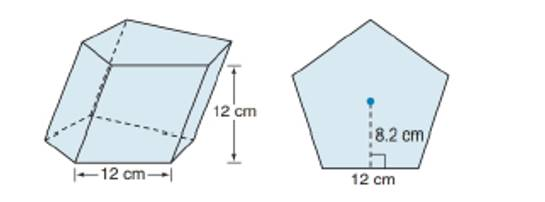Chapter 9.1, Problem 43E### Elementary Geometry for College St...

6th Edition
Daniel C. Alexander + 1 other
ISBN: 9781285195698

#### Solutions

Chapter
Section### Elementary Geometry for College St...

6th Edition
Daniel C. Alexander + 1 other
ISBN: 9781285195698
Textbook Problem
5 views

# For Exercise 43 to 45, consider the oblique regular pentagonal prism shown. Each side of the base measures 12 cm, and the altitude measures 12 cm.Find the lateral area of the prism.(HINT: Each lateral face is a parallelogram.)To determine

To find:

The lateral area of the prism.

Explanation

The lateral area of any solid is the area of all the number of lateral sides that it have, excluding the area of the top and the bottom of the solid.

The lateral area (L) of any solid prison with the perimeter of the base of the prism as (P) and altitude ‘h’ is given by the formula

L=Ph.

Here, P is the perimeter of any one of the base of the prism and which is given by the sum of all the sides of the base.

Calculation:

Given,

The measure of the base edge of the regular pentagonal = 12 cm.

The altitude of the measures = 12 cm.

The lateral area of any prism of altitude ‘h’ and of base perimeter P is given by,

L=hP

Here, we have

h=12 cm

### Still sussing out bartleby?

Check out a sample textbook solution.

See a sample solution

#### The Solution to Your Study Problems

Bartleby provides explanations to thousands of textbook problems written by our experts, many with advanced degrees!

Get Started

#### In Problems 19-44, factor completely. 41.

Mathematical Applications for the Management, Life, and Social Sciences

#### In Exercises 6372, evaluate the expression. 63. |6 + 2|

Applied Calculus for the Managerial, Life, and Social Sciences: A Brief Approach

#### 01e2xdx= a) 2e2 1 b) `e2 1 c) 12e2 d) 12(e21)

Study Guide for Stewart's Single Variable Calculus: Early Transcendentals, 8th